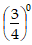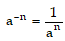# Positive And Negative Exponents Of Real Number

## Number system of Class 9

### EXPONENTS OF REAL NUMBER

#### Positive Integral Power :

For any real number a and a positive integer ‘n’ we define an as :

an = a × a × a × ............... x a (n times)

an is called then nth power of a. The real number ‘a’ is called the base and ‘n’ is called the exponent of the nth power of a.

e.g.  23 = 2 × 2 × 2 = 8

NOTE : For any non-zero real number ‘a’ we define ao = 1.

e.g. thus,3o = 1, 5o,= 1 and so on.

#### Negative Integral Power :

For any non-zero real number ‘a’ and a positive integer ‘n’ we defineThus we have defined an find all integral values of n, positive, zero or negative. an is called the nth power of a.